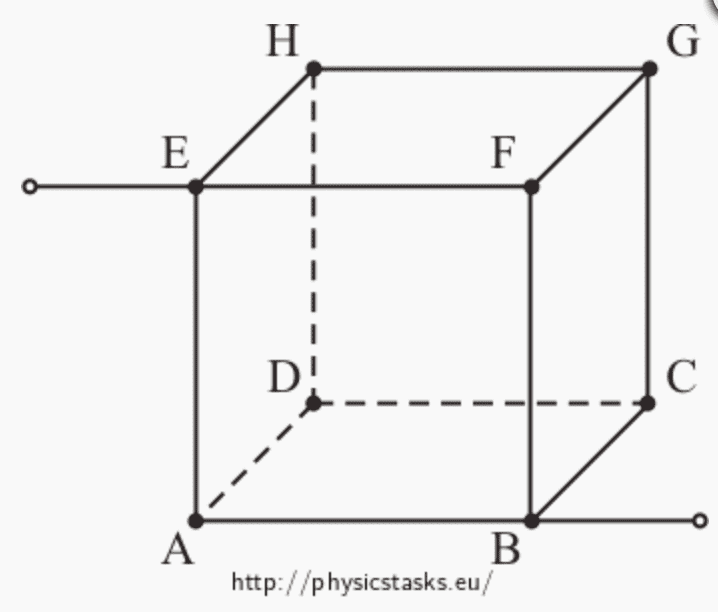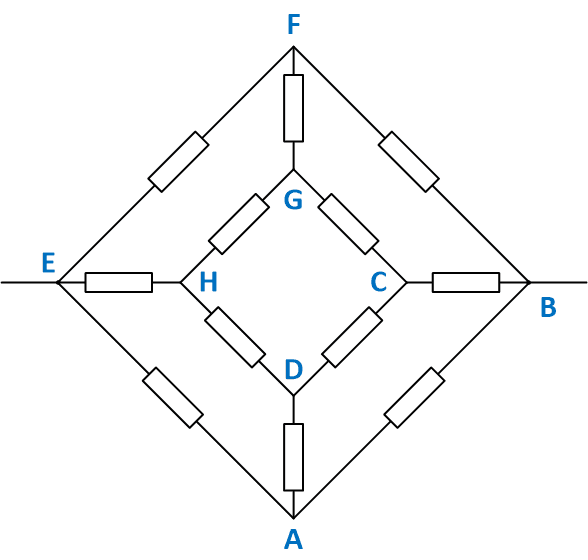# Current in a cube resistor

• johnio09

#### johnio09

Homework Statement
I am given this cube of resistors, each with the same resistance of 4 Ω. The current that enters at E and leaves B is 6 A. I have found that the equivalent resistance is 3 Ω. However, I am unsure of how to find the current through the resistor between E and F.

Note that there are resistors between each of the two letters in the image.
Relevant Equations
Kirchoff's LawsI would think that it is 2 A (6/3 because there are three wires the current can go to), but this does not seem to be correct.

Calculate the voltage across E and B. What do you think the voltage at F is then? (Think symmetry.)

I would think that it is 2 A (6/3 because there are three wires the current can go to), but this does not seem to be correct.
Probably because guessing is no substitution for writing loop or node equations.

because there are three wires the current can go to
But they are not equivalent. Having fixed B and E as the pair of interest, EA and EF are equivalent, but a path starting EH has further to go.
You do not say how you found the resistance to be 3Ω. Using the method that is obvious to me, it easy to determine what fraction of the current flows in each path.

Yes. Guessing is pointless.

OTOH, looking for symmetry to simplify things before you write the equations makes problems like this a lot simpler.

It's hard for me to think in 3D for this stuff. If the answer isn't obvious, the first thing I'll do is redraw the schematic as a planer (2D) network making it as symmetric as possible.

Frankly, I really dislike this sort of quiz question. There's no reason this needs to be a cube, it's an unnecessary trick IMO. In fact the planer diagram doesn't even have wires that cross. In my 30+ years as a practicing analog EE, I've drawn and solved lots of networks. I don't ever recall 3D geometry being a part of a circuit network problem. Yes, you can invent some, they do exist, but it's a rare thing that a good EE can solve somehow, even without practice.

This is a great example of the sort of electronics question you'll see in a physics class, but probably never in an EE class.

Yes. Guessing is pointless.

OTOH, looking for symmetry to simplify things before you write the equations makes problems like this a lot simpler.
Agree ! Old Dutch expression: laziness makes inventive...

It's hard for me to think in 3D for this stuff. If the answer isn't obvious, the first thing I'll do is redraw the schematic as a planer (2D) network making it as symmetric as possible.
I think that's the core of this exercise. More brain gymnastics than anything else. And mentally flattening the cube is so much fun ! I can't resist to post this spoiler:Frankly, I really dislike this sort of quiz question. There's no reason this needs to be a cube, it's an unnecessary trick IMO. In fact the planer diagram doesn't even have wires that cross. In my 30+ years as a practicing analog EE, I've drawn and solved lots of networks. I don't ever recall 3D geometry being a part of a circuit network problem. Yes, you can invent some, they do exist, but it's a rare thing that a good EE can solve somehow, even without practice.

This is a great example of the sort of electronics question you'll see in a physics class, but probably never in an EE class.
Again: agree. But the world is so much greater than just EE##\ ##

•phinds and DaveE
Also note that resistors that have no voltage drop across them also have no current through them and can effectively be removed from the network for analysis. It does make a different network, but it has the same solution. This is a problem that can be solved with no equations.